# CW structure of spheres

This article describes how spheres can be realized as CW-spaces, i.e., how to construct a CW-complex whose underlying topological space is a sphere.

## Description of cells and attaching maps

### The case$n = 0$

The space here is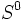$S^0$, a discrete two-point space. There is an obvious and unique CW-structure for this space: two 0-cells, and no other cells.

### The case$n > 0$

The sphere$S^n$ has one 0-cell and one$n$-cell. The The 0-cell gives a point. The attaching map for the boundary of the$n$-cell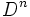$D^n$ sends its boundary (homeomorphic to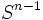$S^{n-1}$) to the 0-cell point. Specifically, we are using the fact that$S^n \cong D^n/\partial D^n$.

## Cellular chain complex and cellular homology

Any CW structure on a topological space provides a cellular filtration relative to the empty space. The corresponding cellular chain complex is described below. By excision, the$k^{th}$ cellular chain group is$\mathbb{Z}^d$ where$d$ is the number of$k$-cells.

### The case$n = 0$

In this case, the zeroth chain group is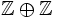$\mathbb{Z} \oplus \mathbb{Z}$ and all higher chain groups are zero. All boundary maps for this chain complex are zero, so the homology groups are the same as the chain groups. In particular,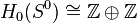$H_0(S^0) \cong \mathbb{Z} \oplus \mathbb{Z}$ and all higher homology groups are zero.

### The case$n > 0$

In this case, the zeroth and$n^{th}$ chain groups are$\mathbb{Z}$ and all other chain groups are zero. All boundary maps for this chain complex are zero, so the homology groups are the same as the chain groups. In particular,$H_0(S^n) \cong H_n(S^n) \cong \mathbb{Z}$ and all other homology groups are zero.

Note that for$n > 1$, the fact that all the chain maps are zero is forced just by looking at the chain groups. This is not the case for$n = 1$, but a careful look at the boundary map from the first to the zeroth chain group reveals that it is zero.